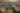# Bayesian inversion of a diffusion model with application to biology

Date July 6, 2021
Authors Jean-Charles Croix, Nicolas Durrande, Mauricio A Alvarez

A common task in experimental sciences is to fit mathematical models to real-world measurements to improve understanding of natural phenomenon (reverse-engineering or inverse modelling). When complex dynamical systems are considered, such as partial differential equations, this task may become challenging or ill-posed.

In this work, a linear parabolic equation is considered as a model for protein transcription from MRNA. The objective is to estimate jointly the differential operator coefficients, namely the rates of diffusion and self-regulation, as well as a functional source.

The recent Bayesian methodology for infinite dimensional inverse problems is applied, providing a unique posterior distribution on the parameter space continuous in the data. This posterior is then summarized using a Maximum a Posteriori estimator. Finally, the theoretical solution is illustrated using a state-of-the-art MCMC.

View the paper

Share
,,